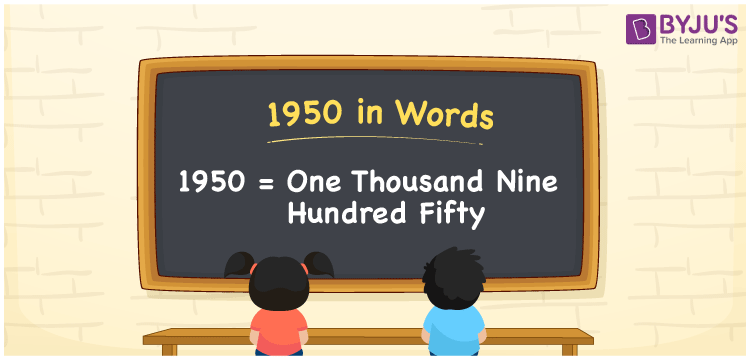# 1950 in Words

1950 in words is written as One thousand nine hundred fifty. In both the International System of Numerals and the Indian System of Numerals, 1950 is written as One thousand nine hundred fifty.

We often use the number 1950 as “1950 applications are approved”. 1950 represents a quantity, “how much of something” or “how many of something”. Hence, the number 1950 is a Cardinal Number.

 1950 in words One thousand nine hundred fifty One thousand nine hundred fifty in Number 1950

## 1950 in English Words

1950 in English words is read as “One thousand nine hundred fifty.”## How to Write 1950 in Words?

To write 1950 in words, we shall use the place value chart. In the place value chart, put 1 in the thousands, 9 in the hundreds, 5 in the tens and 0 in the ones, respectively. Let us make a place value chart to write the number 1950 in words.

 Thousands Hundreds Tens Ones 1 9 5 0

Thus, we can write the expanded form as

1 × Thousand + 9 × Hundred + 5 × Ten + 0 × One

= 1 × 1000 + 9 × 100 + 5 × 10 + 0 × 1

= 1000 + 900 + 50 + 0

= 1950

= One thousand nine hundred fifty.

1950 is the natural number that is succeeded by 1949 and preceded by 1951.

1950 in words – One thousand nine hundred fifty

• Is 1950 an odd number? – No
• Is 1950 an even number? – Yes
• Is 1950 a perfect square number? – No
• Is 1950 a perfect cube number? – No
• Is 1950 a prime number? – No
• Is 1950 a composite number? – Yes

## Frequently Asked Questions on 1950 in Words

Q1

### How to write 1950 in words?

1950 in words is written as One thousand nine hundred fifty.
Q2

### How to write 1950 in words in the International and Indian System of Numerals?

In both, the system of numerals, 1950 in words, is written as One thousand nine hundred fifty.
Q3

### How to write 1950 in a place value chart?

In the place value chart, write 1 in the thousands, 9 in the hundreds, 5 in the tens and 0 in the ones, respectively.